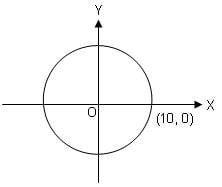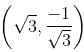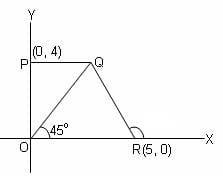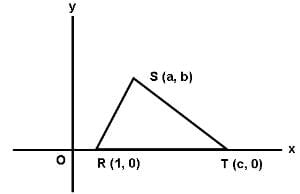CAT  >  Practice Questions Level 2: Coordinate Geometry - 2

# Practice Questions Level 2: Coordinate Geometry - 2 - Notes | Study Level-wise Practice Questions for CAT Preparation - CAT

 1 Crore+ students have signed up on EduRev. Have you?

This EduRev document offers 20 Multiple Choice Questions (MCQs) from the topic Coordinate Geometry (Level - 2). These questions are of Level - 2 difficulty and will assist you in the preparation of CAT & other MBA exams. You can practice/attempt these CAT Multiple Choice Questions (MCQs) and check the explanations for a better understanding of the topic.

Question for Practice Questions Level 2: Coordinate Geometry - 2
Try yourself:What kind of a quadrilateral is formed by the vertices (0, 0), (4, 3), (3, 5) and (-1, 2)?

Question for Practice Questions Level 2: Coordinate Geometry - 2
Try yourself:If a, b, c are in A.P. then the fixed point through which the straight line ax + 2by + c = 0 will always pass, is

Question for Practice Questions Level 2: Coordinate Geometry - 2
Try yourself:In the above figure, if O(0, 0) is the centre of the circle, then which of the following points lies outside the circle?Question for Practice Questions Level 2: Coordinate Geometry - 2
Try yourself:Find the equation of a straight line which contains the pointwith inclination 150o.

Question for Practice Questions Level 2: Coordinate Geometry - 2
Try yourself:Find the point which divides the line joining the points A (1, 3) and B (2, 7) in the ratio of 3 : 4 externally.

Question for Practice Questions Level 2: Coordinate Geometry - 2
Try yourself:If C is the centroid of the triangle PQR and the coordinates of points P, Q, R and C respectively are (x, 1), (0, y), (1/2, 0) and (1/2, 1/3), find the measure of angle PQR.

Question for Practice Questions Level 2: Coordinate Geometry - 2
Try yourself:In the xy-plane, which of the following points is the greatest distance from the origin?

Question for Practice Questions Level 2: Coordinate Geometry - 2
Try yourself:The projection (the foot of perpendicular) from (x, y) on the x-axis is

Question for Practice Questions Level 2: Coordinate Geometry - 2
Try yourself:If a, b and c are the sides of a triangle, and a2 + b2 + c2 = ab + bc + ca, then the triangle is

Question for Practice Questions Level 2: Coordinate Geometry - 2
Try yourself:In the given figure, if PQ is parallel to OR, then what is the area of quadrilateral PQRO?Question for Practice Questions Level 2: Coordinate Geometry - 2
Try yourself:The point that divides the line joining the points (1, 2) and (3, 4) internally in the ratio of 1 : 1 lies in the

Question for Practice Questions Level 2: Coordinate Geometry - 2
Try yourself:The line (1 + K)X + (3 – K)Y = 2(1 + 3K) passes through a fixed point P for any value of K. Find the co-ordinates of P.

Question for Practice Questions Level 2: Coordinate Geometry - 2
Try yourself:The vertices of a square S have coordinates (-1, -2), (-1, 1), (2, 1) and (2, -2). What are the coordinates of the point where the diagonals of S intersect?

Question for Practice Questions Level 2: Coordinate Geometry - 2
Try yourself:In the rectangular coordinate system shown below, the area of triangle RST is _______.Question for Practice Questions Level 2: Coordinate Geometry - 2
Try yourself:The incentre of a triangle formed by the lines y = 0, 3x + 4y = 48 and 3y - 4x = 36 is

Question for Practice Questions Level 2: Coordinate Geometry - 2
Try yourself:Two points (a, 0) and (0, b) are joined by a straight line. Another point on this line is

Question for Practice Questions Level 2: Coordinate Geometry - 2
Try yourself:If the coordinates of the centroid of a triangle are (2/3, 5/3) and the coordinates of two vertices of the triangle are (-1, 0) and (3, 0), then what are the coordinates of the third vertex of the triangle?

Question for Practice Questions Level 2: Coordinate Geometry - 2
Try yourself:A line segment with end points A(-6, 10) and B(3, -8) is divided in the ratio 2 : 7 by a point P. Find the coordinates of point P.

Question for Practice Questions Level 2: Coordinate Geometry - 2
Try yourself:If point (t, 1) is located inside the circle x2 + y2 = 10, then t must lie between

Question for Practice Questions Level 2: Coordinate Geometry - 2
Try yourself:Find the inclination and perpendicular distance of a line represented by (x/2) + (√3y/2) = 12√5 from the origin.

The document Practice Questions Level 2: Coordinate Geometry - 2 - Notes | Study Level-wise Practice Questions for CAT Preparation - CAT is a part of the CAT Course Level-wise Practice Questions for CAT Preparation.
All you need of CAT at this link: CAT

## Level-wise Practice Questions for CAT Preparation

277 docs
 Use Code STAYHOME200 and get INR 200 additional OFF

## Level-wise Practice Questions for CAT Preparation

277 docs

### How to Prepare for CAT

Read our guide to prepare for CAT which is created by Toppers & the best Teachers

Track your progress, build streaks, highlight & save important lessons and more!

,

,

,

,

,

,

,

,

,

,

,

,

,

,

,

,

,

,

,

,

,

;